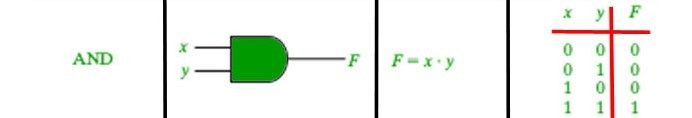Related Articles

# && operator in Java with Examples

• Last Updated : 30 Sep, 2019

&& is a type of Logical Operator and is read as “AND AND” or “Logical AND“. This operator is used to perform “logical AND” operation, i.e. the function similar to AND gate in digital electronics.One thing to keep in mind is the second condition is not evaluated if the first one is false, i.e. it has a short-circuiting effect. Used extensively to test for several conditions for making a decision.

Syntax:

```Condition1 && Condition2

// returns true if both the conditions are true.
```

Below is an example to demonstrate && operator:

Example:

 `// Java program to illustrate``// logical AND operator`` ` `import` `java.util.*;`` ` `public` `class` `operators {``    ``public` `static` `void` `main(String[] args)``    ``{`` ` `        ``int` `num1 = ``10``;``        ``int` `num2 = ``20``;``        ``int` `num3 = ``30``;`` ` `        ``// find the largest number``        ``// using && operator``        ``if` `(num1 >= num2 && num1 >= num3)``            ``System.out.println(``                ``num1``                ``+ ``" is the largest number."``);``        ``else` `if` `(num2 >= num1 && num2 >= num3)``            ``System.out.println(``                ``num2``                ``+ ``" is the largest number."``);``        ``else``            ``System.out.println(``                ``num3``                ``+ ``" is the largest number."``);``    ``}``}`
Output:
```30 is the largest number.
```

Attention reader! Don’t stop learning now. Get hold of all the important Java Foundation and Collections concepts with the Fundamentals of Java and Java Collections Course at a student-friendly price and become industry ready. To complete your preparation from learning a language to DS Algo and many more,  please refer Complete Interview Preparation Course.

My Personal Notes arrow_drop_up